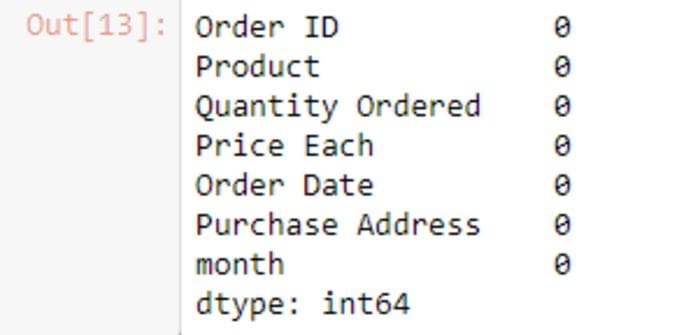# E-commerce Data Analysis for Sales Strategy Using Python

Check out this informative and concise case study applying data analysis using Python to a well-defined e-commerce scenario.

By Juhi Sharma, Product AnalystSource — https://www.wvgazettemail.com/

### Introduction

Kmart is a leading online retailer in the US and as part of their annual sales review meeting, they need to decide on their sales strategy for the year 2020 based on the insights from the sales data in 2019.

Data is related to sales for each month of 2019 and the task is to generate key insights which will help the sales team of Kmart to take some key business decisions towards Fine-tuning their sales strategy.

### Data Understanding

1. Data Belongs to Kmart -a leading online retailer in the US.
2. Time Period — January 2019 — December 2019
3. Unique Product — 19
4. Total Orders — 178437
5. Cities — 9
6. KPI’s — Total Sales, Total Products SoldSource — By Author

### Business Problem Statements

1. What was the best month for sales? How much was earned that month?
2. Which city had the highest number of sales?
3. Recommend the most appropriate time to display advertising to maximize the likelihood of customers buying the products?
4. What products sold the most? Why do you think it sold the most?

### Data Analysis Using Python

1. Loaded Data of each month and made data frame using pandas
2. Concatenated Dataset to make one Dataset for 2019 sales.
3. Treating Null Values and Junk Data.
4. Made a Filtered Dataset after preprocessing data
5. Analysis and answers to business problems. (visualizations using matplot and seaborn library)

### 1. Importing Libraries

``import pandas as pd``

### 2. Loading Dataset and making Dataframes

``````df1=pd.read_csv("Sales_January_2019.csv")
df1["month"]="Jan"
df2["month"]="feb"
df3["month"]="mar"
df4["month"]="apr"
df5["month"]="may"
df6["month"]="june"
df7["month"]="july"
df8["month"]="aug"
df9["month"]="sep"
df10["month"]="oct"
df11["month"]="nov"
df12["month"]="dec"list=[df1,df2,df3,df4,df5,df6,df7,df8,df9,df10,df11,df12]``````

### 3. The shape of each month’s dataset

``````for i in list:
print(i.shape)``````Source- By Author

### 4. Concatenating dataset

``frame=pd.concat(list)``Source- By Author

### 5. Columns of Final Dataset

``frame.columns``Source-By Author

### 6. Dataframe Information

``frame.info()``Source-By Author

### 7. Null values in the dataset

``frame.isnull().sum() # there are 545 null values in each column except month``Source-By Author

``(frame.isnull().sum().sum())/len(frame)*100  # we have 1.75 percent null values , so we can drop them``Source-By Author

### 8. Dropping Null Values

``````frame=frame.dropna()
frame.isnull().sum()``````Source-By Author

### 9. Removing Junk Data

we observed that there are 355 columns in which values in rows are the same as the header. so making a new data frame where these values will be excluded.

``frame[frame['Quantity Ordered'] == "Quantity Ordered"]````````df_filtered = frame[frame['Quantity Ordered'] != "Quantity Ordered"]
df_filtered.shape``````Source-By Author

### Solutions to Business Problems

Q 1. What was the best month for sales? How much was earned that month?

``````df_filtered["Quantity Ordered"]=df_filtered["Quantity Ordered"].astype("float")
df_filtered["Price Each"]=df_filtered["Price Each"].astype("float")# Creating Sales Column By multiplying Quantity Ordered and Price of Each Productdf_filtered["sales"]=df_filtered["Quantity Ordered"]*df_filtered["Price Each"]``````Source-By Author

``````month=["dec","oct","apr","nov","may","mar","july","june","aug",'feb',"sep","jan"]
df["month"]=monthfrom matplotlib import pyplot as plt
a4_dims = (11.7, 8.27)
fig, ax = pyplot.subplots(figsize=a4_dims)
import seaborn as sns
sns.barplot(x = "sales",
y = "month",
data = df)
plt.title("Month wise Sale")
plt.show()``````Source-By Author

The best Month for sales was DECEMBER.

Total sales in December is \$ 4619297.

Q 2. Which city had the highest number of sales?

``````dftemp = df_filtered
list_city = []
for i in dftemp['Purchase Address']:
list_city.append(i.split(","))
dftemp['City'] = list_citySource-By Author

``````df_city=df_filtered.groupby(["City"])['sales'].sum().sort_values(ascending=False)
df_city=df_city.to_frame()
df_city``````Source-By Author

``````city=["San Francisco","Los Angeles","New York City","Boston","Atlanta","Dallas","Seattle","Portland","Austin"]
df_city["city"]=cityfrom matplotlib import pyplot
a4_dims = (11.7, 8.27)
fig, ax = pyplot.subplots(figsize=a4_dims)
sns.barplot(x = "sales",
y = "city",
data = df_city)
plt.title("City wise Sales")
plt.show()``````Source-By Author

San Francisco has the highest sales f around \$8262204.

Q 3 What products sold the most?

``````print(df_filtered["Product"].unique())
print(df_filtered["Product"].nunique())``````source- By Author

``````df_p=df_filtered.groupby(['Product'])['Quantity Ordered'].sum().sort_values(ascending=False).head()
df_p=df_p.to_frame()
df_p``````Source-By Author

``````product=["AAA Batteries (4-pack)","AA Batteries (4-pack)","USB-C Charging Cable","Lightning Charging Cable","Wired Headphones"]
df_p["Product"]=productfrom matplotlib import pyplot
a4_dims = (11.7, 8.27)
fig, ax = pyplot.subplots(figsize=a4_dims)
sns.barplot(x = "Quantity Ordered",
y = "Product",
data = df_p)
plt.title("Prouct and Quantity Ordered")
plt.show()``````Source-By Author

31017.0 quantity of AAA Batteries (4-pack) is sold in a year. It is sold maximum because it is the cheapest product.

Q 4 Recommend the most appropriate time to display advertising to maximize the likelihood of customers buying the products?

``````dftime = df_filtered
list_time = []
for i in dftime['Order Date']:
list_time.append(i.split(" "))
dftime['Time'] = list_timeSource-By Author

``````df_t=df_filtered.groupby(['Time'])['sales'].sum().sort_values(ascending=False).head()
df_t=df_t.to_frame()
df_t``````Source-By Author

``df_t.columns``Source -By Author

### Before You Go

Thanks for reading! If you want to get in touch with me, feel free to reach me at jsc1534@gmail.com or my LinkedIn Profile. Also, you can find the code for this article and some really useful data science projects on my GitHub account.

Bio: Juhi Sharma (Medium, GitHub) has 2+ years of work experience as an Analyst with the role of Project Management, Business Analysis, and Client Handling. Currently, Juhi is working as a Data Analyst for a Product Company. Juhi has hands-on experience in analyzing datasets, creating machine learning and deep learning models. Juhi is passionate about solving business problems with data-driven approaches.

Original. Reposted with permission.

Related:

By subscribing you accept KDnuggets Privacy Policy

By subscribing you accept KDnuggets Privacy Policy

By subscribing you accept KDnuggets Privacy Policy